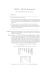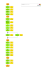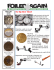# Slides - faculty.ucmerced.edu

## Transcription

Slides - faculty.ucmerced.edu
```CSE 115 / ENGR 160
Spring 2015
Lecture 14
Announcements and Assignments
• Check course website for reading schedule
• HW #6 due Wed. Mar. 18 in class
• Quiz #2 on Wed. Apr. 1
– More on next slide
Quizzes
• Second quiz is Wed. Apr. 1.
• During last ~30 minutes of lecture.
• Covers sections 2.3-2.6 and 3.1:
– Lectures 10 through 14.
– HWs #5 and #6.
• CLOSED BOOK & CLOSED NOTES.
Today
• 3.1 Algorithms (continued)
Midterm #1
• Maximum number of points: 33
• Max. score: 32 (3 people)
• Average: 25.2 (76.3%)
Histogram
16
14
Frequency
12
10
8
6
4
2
0
16.5
19.8
23.1
26.4
29.7
More
•
•
•
•
•
•
>90% (29.7): 8
>80% (26.4): 12
>70% (23.1): 15
>60% (19.8): 12
>50% (16.5): 3
<50% (16.5): 1
Specifying Algorithms
 Algorithms can be specified in different ways. Their steps can 



be described in English or in pseudocode.
Pseudocode is an intermediate step between an English language description of the steps and a coding of these steps using a programming language. The form of pseudocode we use is specified in Appendix . It uses some of the structures found in popular languages such as C++ and Java.
Programmers can use the description of an algorithm in pseudocode to construct a program in a particular language. Pseudocode helps us analyze the time required to solve a problem using an algorithm, independent of the actual programming language used to implement algorithm. 8
Properties of Algorithms
 Input: An algorithm has input values from a specified set.
 Output: From the input values, the algorithm produces the 



output values from a specified set. The output values are the solution.
Correctness: An algorithm should produce the correct output values for each set of input values.
Finiteness: An algorithm should produce the output after a finite number of steps for any input.
Effectiveness: It must be possible to perform each step of the algorithm correctly and in a finite amount of time.
Generality: The algorithm should work for all problems of the desired form.
9
Finding the Maximum Element in a Finite Sequence
 The algorithm in pseudocode:
procedure max(a1, a2, …., an: integers)
max := a1
for i := to n
if max < ai then max := ai
return max{max is the largest element}
 Does this algorithm have all the properties listed on the previous slide? 10
Some Example Algorithm Problems
 Three classes of problems will be studied in this section.
1. Searching Problems: finding the position of a particular element in a list.
2. Sorting Problems: putting the elements of a list into increasing order.
3. Optimization Problems: determining the optimal value (maximum or minimum) of a particular quantity over all possible inputs.
11
Searching Problems
Definition: The general searching problem is to locate an element x in the list of distinct elements a1,a2,...,an, or determine that it is not in the list.
 The solution to a searching problem is the location of the term in the list that equals x (that is, i is the solution if x = ai) or if x is not in the list.
 For example, a library might want to check to see if a patron is on a list of those with overdue books before allowing him/her to checkout another book.
 We will study two different searching algorithms: linear search and binary search.
12
Linear Search Algorithm
 The linear search algorithm locates an item in a list by examining elements in the sequence one at a time, starting at the beginning.
First compare x to a1. If they are equal, return the position 1.
If not, try a2. If x = a2, return the position 2.
Keep going, and if no match is found when the entire list is scanned, return 0.
procedure linear search(x:integer, a1, a2, …,an: distinct integers)
i := 1
while (i n and x ≠ ai)
i := i + 1
if i n then location := i
else location := 0
return location{location is the subscript of the term that equals x, or is 0 if x is not found}
 Why is this referred to as a “linear” algorithm?
13
Binary Search
 Assume the input is a list of items in increasing order.
 The algorithm begins by comparing the element to be found with the middle element.  If the middle element is lower, the search proceeds with the upper half of the list.
 If it is not lower, the search proceeds with the lower half of the list (through the middle position).
 Repeat this process until we have a list of size 1.
 If the element we are looking for is equal to the element in the list, the position is returned.
 Otherwise, 0 is returned to indicate that the element was not found.  In Section 3.3, we show that the binary search algorithm is much more efficient than linear search.
14
Binary Search
 Here is the pseudocode for the binary search algorithm: procedure binary search(x: integer, a1,a2,…, an: increasing integers)
i := 1 {i is the left endpoint of interval}
j := n {j is right endpoint of interval}
while i < j
m := (i + j)/2
if x > am then i := m + 1
else j := m
if x = ai then location := i
else location := 0
return location{location is the subscript i of the term ai equal to x, or 0 if x is not found} 15
Example: The steps taken by a binary search for 19 in the list:
1235678101213151618192022
1. Thelisthas16elements,sothemidpointis8.Thevalueinthe8th positionis
10.Since19 10,furthersearchisrestrictedtopositions9 through16.
123567810 1213151618192022
2. Themidpointofthelist positions9through16 isnowthe12th position
withavalueof16.Since19 16,furthersearchisrestrictedtothe13th
positionandabove.
123567810 1213151618192022
3. Themidpointofthecurrentlistisnowthe14th positionwithavalueof19.
Since19≯19,furthersearchisrestrictedtotheportionfromthe13th
throughthe14th positions.
123567810 1213151618192022
4. Themidpointofthecurrentlistisnowthe13th positionwithavalueof18.
Since19 18,searchisrestrictedtotheportionfromthe18th position
throughthe18th.
123567810 1213151618 192022
5. Nowthelisthasasingleelementandtheloopends.Since19 19,thelocation
16isreturned.
16
Sorting
 To sort the elements of a list is to put them in increasing order (numerical order, alphabetic, and so on).
 Sorting is an important problem because:
 A nontrivial percentage of all computing resources are devoted to sorting different kinds of lists, especially applications involving large databases of information that need to be presented in a particular order.
 An amazing number of fundamentally different algorithms have been invented for sorting.
 Sorting algorithms are useful to illustrate the basic notions of computer science.
 A variety of sorting algorithms are studied in this book; binary, insertion, bubble, selection, merge, quick, and tournament.
 In Section 3.3, we’ll study the amount of time required to sort a 17
list using the sorting algorithms covered in this section.
Bubble Sort
 Bubble sort makes multiple passes through a list. Every pair of elements that are found to be out of order are interchanged.
procedure bubblesort(a1,…,an: real numbers with n ≥ )
for i := to n
for j := to n i
if aj >aj+1 then interchange aj and aj+1
{a1,…,an is now in increasing order}
18
Bubble Sort
Example: Show the steps of bubble sort with 32415.
 Attheendofthefirstpass,thelargestelementhasbeenputinto
thecorrectposition.
 Attheendofthesecondpass,the2nd largestelementhasbeen
putintothecorrectposition.
19
correctposition.
Insertion Sort
 Insertion sort begins with the nd
element. It compares the nd element with the st and puts it before the first if it is not larger.
procedure insertion sort
• Next the 3rd element is put (a1,…,an: real numbers with n ≥ 2)
into the correct position for j := 2 to n
among the first 3 i := 1
elements. while aj > ai
• In each subsequent pass, i := i + 1
st
the n+ element is put m := aj
into its correct position for k := 0 to j i 1
among the first n+
aj‐k := aj‐k‐1
elements.
ai := m
• Linear search is used to find the correct position. {Now a1,…,an is in increasing order}
20
Insertion Sort
Example: Show all the steps of insertion sort with the input: first two positions are interchanged)
element remains in its position)
fourth is placed at beginning)
element remains in its position)
21
Greedy Algorithms
 Optimization problems minimize or maximize some parameter over all possible inputs.
 Among the many optimization problems we will study are:
 Finding a route between multiple cities with the smallest total mileage.
 Determining how to encode messages using the fewest possible bits.
 Optimization problems can often be solved using a greedy algorithm, which makes the “best” choice at each step. Making the “best choice” at each step does not necessarily produce an optimal solution to the overall problem, but in many instances, it does.  After specifying what the “best choice” at each step is, we try to prove that this approach always produces an optimal solution, or find a counterexample to show that it does not.
 The greedy approach to solving problems is an example of an algorithmic paradigm, which is a general approach for designing an algorithm. We return to algorithmic paradigms in Section 3.3.
22
Greedy Algorithms: Making Change
Example: Design a greedy algorithm for making change (in U.S. money) of n cents with the following coins: quarters ( cents), dimes ( cents), nickels ( cents), and pennies ( cent), using the least total number of coins.
Idea: At each step choose the coin with the largest possible value that does not exceed the amount of change left.
1.
2.
3.
4.
If n = 67 cents, first choose a quarter leaving 67 25 42 cents.Thenchooseanotherquarterleaving
42 25 17cents.
Thenchoose1dime,leaving17 10 7cents.
Choose1nickel,leaving7– 5 2cents.
Chooseapenny,leavingonecent.Chooseanotherpenny
leaving0cents.
23
Greedy Change‐Making Algorithm
Solution: Greedy change‐making algorithm for n cents. The algorithm works with any coin denominations c1, c2, …, cr .
procedure change(c1, c2, …, cr: values of coins, where c1>c2> … >cr , n: a positive integer)
for i := 1tor
di := 0 di counts the coins of denomination ci] while n ci
di := di + 1 addacoinofdenominationci
n n ‐ ci
di counts the coins of denomination ci]
 For the example of U.S. currency, we may have quarters, dimes, nickels, and pennies, with c1 = 25, c2 = 10,c3 = 5,andc4 = 1.
 IsitoptimalforU.S.currency? optimal resultsinfewestcoins
 Isitoptimalforalldenominations?
24
Proving Optimality for U.S. Coins
 Show that the change making algorithm for U.S. coins is optimal.
Lemma 1: If n is a positive integer, then n cents in change using quarters, dimes, nickels, and pennies, using the fewest coins possible has at most 2dimes, 1 nickel, 4pennies, and cannot have 2 dimes and a nickel. The total amount of change in dimes, nickels, and pennies must not exceed 24 cents.
 If we had 3 dimes, we could replace them with a quarter and a nickel.  If we had 2 nickels, we could replace them with 1 dime.
 If we had 5 pennies, we could replace them with a nickel.
 If we had 2 dimes and 1 nickel, we could replace them with a quarter.
 The allowable combinations have a maximum value of 24 cents; 2
25
dimes and 4 pennies. Proving Optimality for U.S. Coins
Theorem: The greedy change‐making algorithm for U.S. coins produces change using the fewest coins possible.
1. Assumethereisapositiveintegern suchthatchangecanbe
withafewertotalnumberofcoinsthangivenbythealgorithm.
2. Then,q̍
q whereq̍ isthenumberofquartersusedinthis
optimalwayandq isthenumberofquartersinthegreedy
algorithm’ssolution.ButthisisnotpossiblebyLemma1,since
thevalueofthecoinsotherthanquarterscannotbegreater
than24cents.
3. Similarly,byLemma1,thetwoalgorithmsmusthavethesame
numberofdimes,nickels,andpennies.
26
Greedy Change‐Making Algorithm  Optimality depends on the denominations available.
 For U.S. coins, optimality still holds if we add half dollar coins ( cents) and dollar coins (
cents).
 But if we allow only quarters ( cents), dimes (
cents), and pennies ( cent), the algorithm no longer produces the minimum number of coins.
 Consider the example of cents. The optimal number of coins is , i.e., dimes and penny. What does the algorithm output?
27
Greedy Scheduling
Example: We have a group of proposed talks with start and end times. Construct a greedy algorithm to schedule as many as possible in a lecture hall, under the following assumptions:
 When a talk starts, it continues till the end.
 No two talks can occur at the same time.
 A talk can begin at the same time that another ends.
 Once we have selected some of the talks, we cannot add a talk which is incompatible with those already selected because it overlaps at least one of these previously selected talks.
 How should we make the “best choice” at each step of the algorithm? That is, which talk do we pick?
 The talk that starts earliest among those compatible with already chosen talks?
 The talk that is shortest among those already compatible?
 The talk that ends earliest among those compatible with 28
Greedy Scheduling
 Picking the shortest talk doesn’t work.
Start:8:00AM
Talk 1
End :9:45 AM
Start:9:00AM
Talk 2
Start:9:45AM
End:10:00AM
Talk 3
End:11:00AM
 Can you find a counterexample here?
 But picking the one that ends soonest does work. The algorithm is specified on the next page. 29
Greedy Scheduling algorithm
Solution: At each step, choose the talks with the earliest ending time among the talks compatible with those selected.
procedure schedule(s1 s2 … sn : start times; e1,e2 ,…,en :
end times)
sort talks by finish time and reorder so that e1 e2 … en S := ∅
for j := 1ton
if talk j is compatible with S then S := S ∪{talk j}
return S Sisthesetoftalksscheduled
 Will be proven correct by induction in Chapter 5.
30
```

### CMSC351 - Fall 2014, Homework #4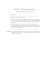### HERE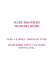### For women, union jobs eliminate the pay gap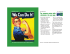### CS2311 Computer Programming, (14-15, Sem. A)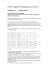### Shove ha`penny - North Road Community Primary School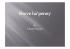### CMSC351 - Fall 2014, Homework #4